# Logical quantifier facts for kids

Kids Encyclopedia Facts

In logic, a quantifier is a way to state that a certain number of elements fulfill some criteria. For example, every natural number has another natural number larger than it. In this example, the word "every" is a quantifier. Therefore, the sentence "every natural number has another natural number larger than it" is a quantified expression.

Quantifiers and quantified expressions are a useful part of formal languages. They are useful because they let rigorous statements claim how widespread a criteria is. Two basic kinds of quantifiers used in predicate logic are universal and existential quantifiers. A universal quantifier states that all the elements considered fulfill the criteria. The universal quantifier is symbolized with "∀", an upside down "A", to stand for "all". An existence quantifier (symbolized with "∃") states that at least one element considered fits the criteria. The existential quantifier is symbolized with "∃", a backwards "E", to stand for "exists".

Quantifiers are also used in natural languages. Examples of quantifiers in English include for all, for some, many, few, a lot, and no.

## Mathematics

This statement is infinitely long:

1 · 2 = 1 + 1, and 2 · 2 = 2 + 2, and 3 · 2 = 3 + 3, ..., and 100 · 2 = 100 + 100, and ..., etc.

This is a problem for formal languages, since a formal statement needs to be finite in length. These problems can be avoided by using universal quantification. This results in the following compact statement:

For each natural number n, n · 2 = n + n.

In the same way, we can shorten an infinite sequence of statements joined by or such as:

1 is equal to 5 + 5, or 2 is equal to 5 + 5, or 3 is equal to 5 + 5, ... , or 100 is equal to 5 + 5, or ..., etc.

by rewriting it using existential quantification:

For at least one natural number n, n is equal to 5+5.

## Notation

The two quantifiers most widely used are the universal quantifier and the existence quantifier.

The universal quantifier is used to claim that for elements in a set, the elements all match some criteria. Usually, this statement "for all elements" is shortened to an "A" flipped upside down, which is "∀".

The existential quantifier is used to claim that for elements in a set, there exists at least one element that matches some criteria. Usually, this statement "there exists an element" is shortened to an "E" flipped upside down, which is "∃".

English statements can be often rewritten using symbols, predicates representing criteria, and quantifiers. One example is "Each of Peter's friends either likes to dance or likes to go to the beach". Let X be the set of all Peter's friends. Let P(x) be the predicate "x likes to dance". Let Q(x) be the predicate "x likes to go to the beach". We can rewrite the example using formal notation as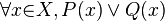$\forall{x}{\in}X, P(x) \or Q(x)$. The statement can be read as "For every x that is a member of X, P applies to x or Q applies to x."

There are other ways to use quantifiers in formal language. Each of the following statements below says the same thing as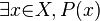$\exists{x}{\in}X, P(x)$:

•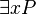$\exists{x} P$
•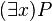$(\exists{x}) P$
•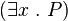$(\exists x \ . \ P)$
•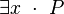$\exists x \ \cdot \ P$
•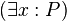$(\exists x : P)$
•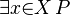$\exists{x}{\in}X \, P$
•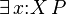$\exists\, x{:}X \, P$

There are a few more ways to represent the universal quantifier:

•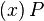$(x) \, P$
•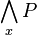$\bigwedge_{x} P$

Several statements above explicitly include X, the set of elements that the quantifier applies to. This set of elements is also known as the range of quantification, or the universe of discourse. Some of the statements above do not include such a set. In this case, the set will have to be specified before the statement. For example, "x is an apple" must be stated before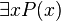$\exists{x} P(x)$. In this case, we are making a statement that at least one apple fits the predicate P.

Using quantifiers formally does not require using the symbol x. In fact, other symbols, such as y and z, can also be used. However, one must be careful not to use the same symbol to refer to two different things.

## Nesting

It is important to put quantifiers in the right order. Below is an example of an English sentence showing how meaning changes with order:

For every natural number n, there exists a natural number s such that s = n2.

This statement is true. It states that every natural number has a square. However, if we turn the order of the quantifiers around:

There exists a natural number s, such that for every natural number n, s = n2.

then the statement is false. It claims that there is one natural number s that is the square of every natural number.

In certain circumstances, changing the order of quantifiers does not change the meaning of the statement. For instance:

There exists a natural number x, and there exists a natural number y such that x = y2.

## Other quantifiers

There are also less common quantifiers used by mathematicians.

An example is the solution quantifier. It is used to state which elements solve a particular equation. The solution quantifier is represented by a § (section sign). For example, the following statement claims the squares of 0, 1 and 2 are smaller than 4.  :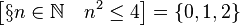$\left [ \S n \in \mathbb{N} \quad n^2 \leq 4 \right ] = \left\{0, 1, 2\right\}$

Other quantifiers include:

• There are many elements such that...
• There are few elements such that...
• There are infinitely many elements such that...
• For all but finitely many elements... (sometimes expressed as "for almost all elements...").
• There are uncountable many elements such that...
• For all but countably many elements...

## History

Term logic was developed by Aristotle. It was an early form of logic, and included quantification. The use of quantification was closer to that of natural language. This meant that statements in term logic with quantifiers were less suited for formal analysis. Term logic included quantifiers for All, Some and No (none) in 4th century BC.

In 1879, Gottlob Frege created a notation for universal quantification. Unlike the modern notation, he would represent a universal quantification by writing a variable over a dimple in an otherwise straight line. Frege did not create a notation for existential quantification. Instead, he combined universal quantification and a number of negations to make an equivalent statement. Frege's use of quantification was not widely known until Bertrand Russell's 1903 Principles of Mathematics.

In 1885, Charles Sanders Peirce and his student Oscar Howard Mitchell also created a notation for universal and existential quantifiers. They wrote Πx and Σx where we now write ∀x and ∃x. Pierce's notation was used by many mathematicians into the 1950s.

In 1897, William Ernest Johnson and Giuseppe Peano created another notation for universal and existential quantification. They were influenced by Pierce's previous quantification notation. Johnson and Peano used the simple (x) for universal quantification, and ∃x for existential quantification. Peano's influence on math spread this notation across Europe.

In 1935, Gerhard Gentzen created the ∀ symbol for universal quantification. It was not widely used until the 1960s.

## Images for kidsLogical quantifier Facts for Kids. Kiddle Encyclopedia.